# Change cell reference in formulas to range names in Excel

If we have previously defined names for the cell reference, then the cell reference in the formula will change to their defined names automatically. This is something that we are familiar with. However, if we establish formulae for the cell reference before defining names, the references to the cells will not be updated to reflect their newly defined names.

## Change Cell reference in formulas to range names in Excel

Let’s understand step by step with an example.

Step 1

At first, create a sample data as shown in following screenshot.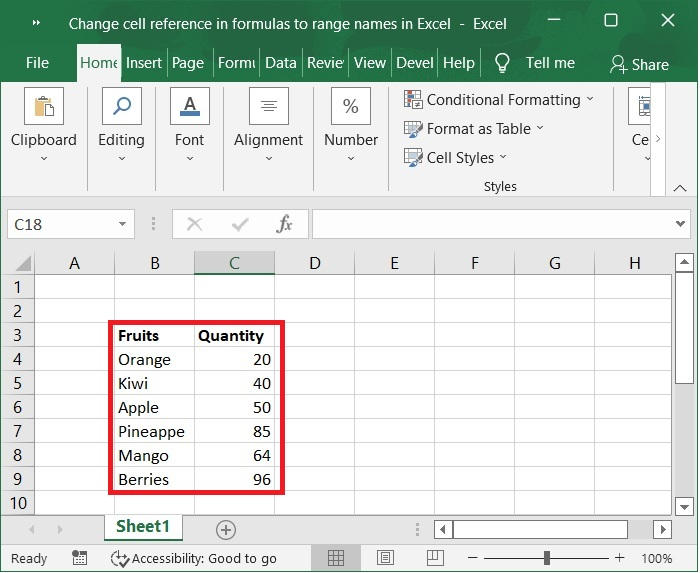Step 2

Next, select the range C4:C9 and click on the Formulas tab and go to the Defined Names group.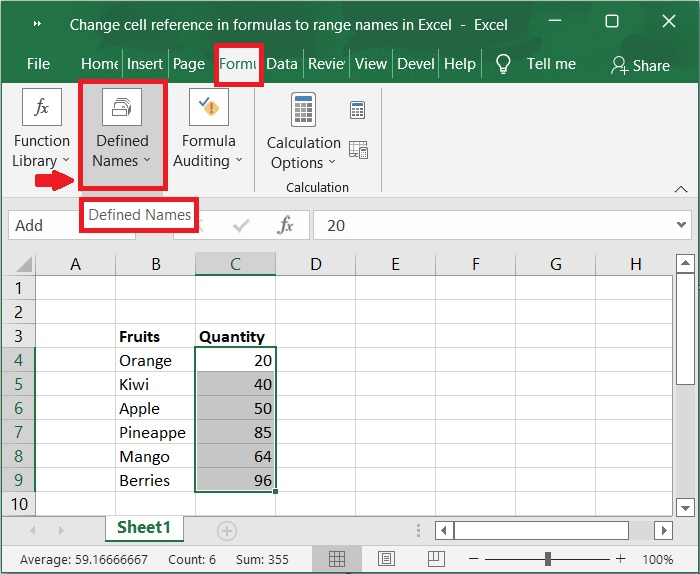Step 3

Now, click the arrow next to the Define Name button and choose Define Names from the drop-down menu that appears.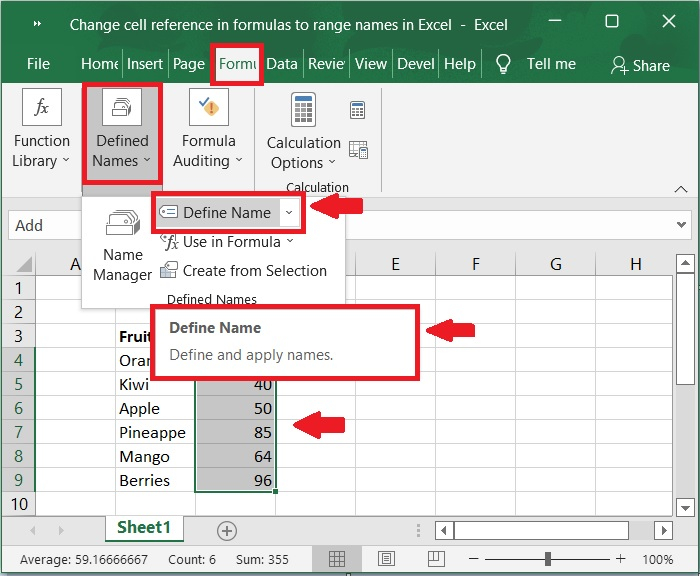Step 4

Now, give any name to that selected range. From the below screenshot we can observe that “add” is the name given to the range C4:C9.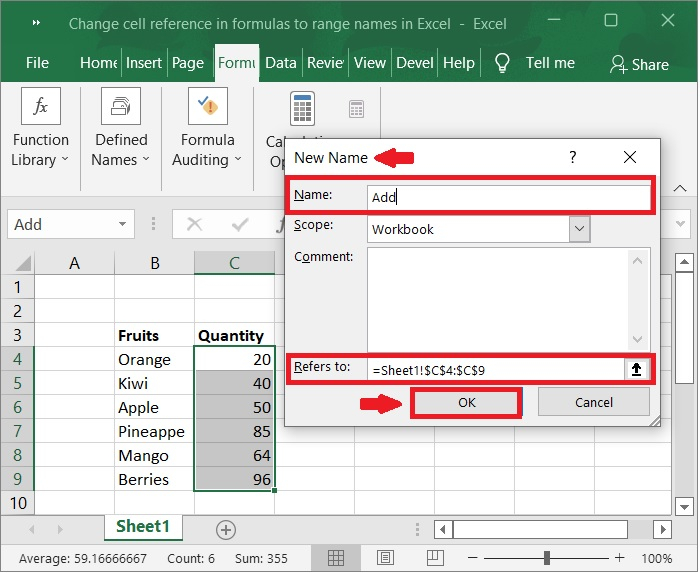Step 5

We can use the defined names in the formulas instead of writing the cell range in formula. Below are the examples showing the formulas with cell ranges and using the defined name in formula.

Formula with cell reference

=sum(C4:C9)


Formula with Names

=sum(add)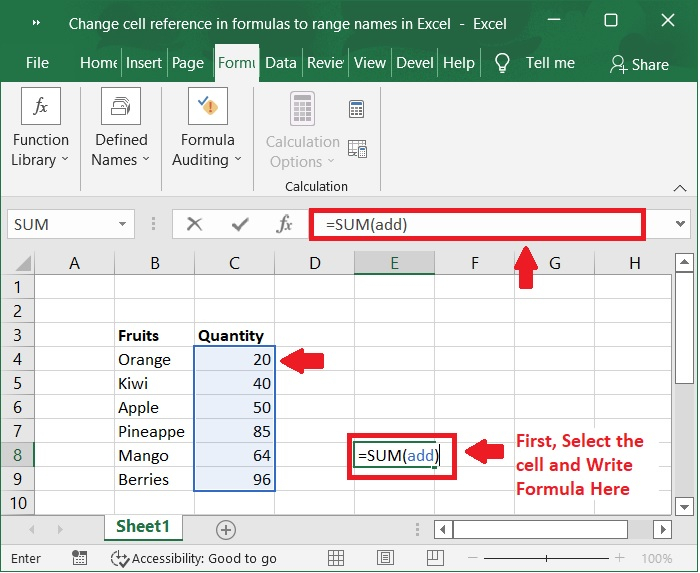Step 6

Now, the sum of the selected named range values is calculated and displayed in the cell.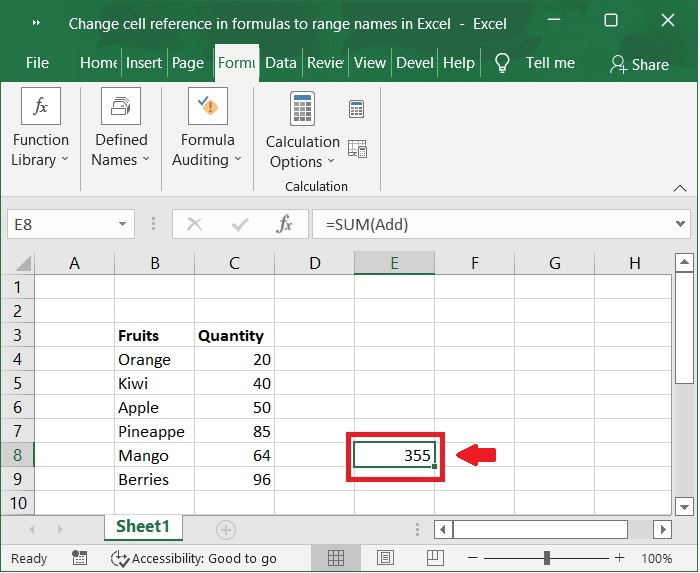### Example 2

Let's take another example.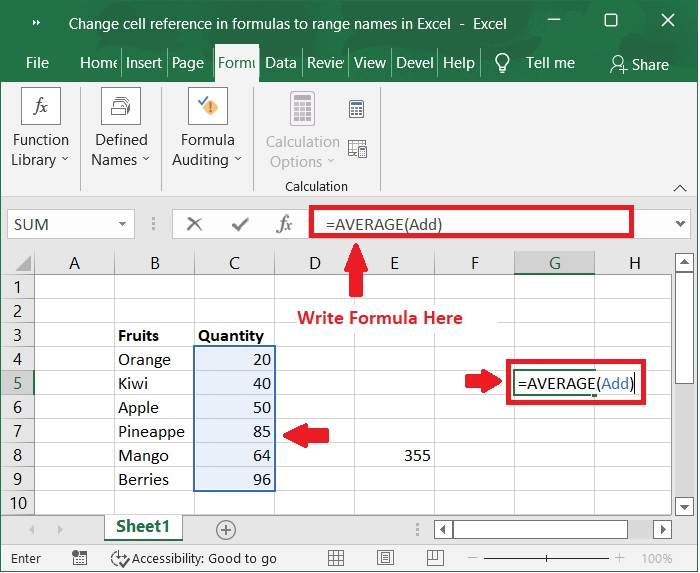Step 7

In the following screenshot, the average of defined named range is calculated and displayed in the cell.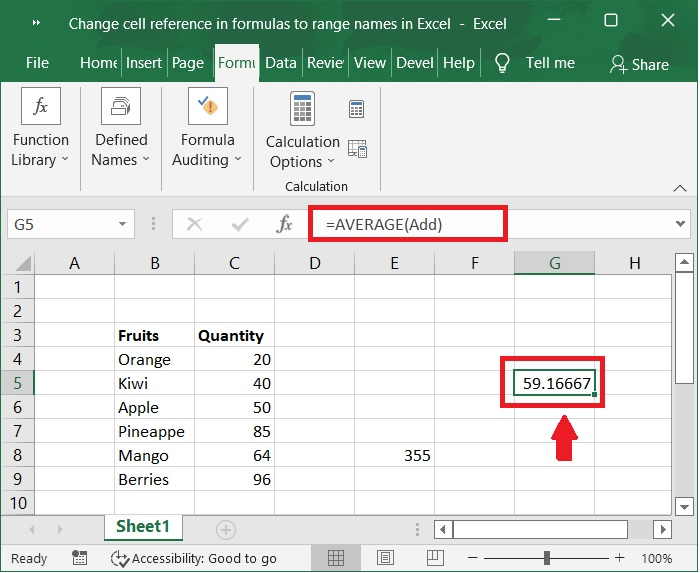## Conclusion

Named ranges in Excel act as a temporary table; we can use the named range anywhere in an Excel workbook. In this tutorial, we explained how you can change the cell reference in formulas to named range.